This article was published as a part of the Data Science Blogathon.

## What is Correlation?

Correlation is used to find the relationship between two variables which is important in real life because we can predict the value of one variable with the help of other variables, who is being correlated with it. It is a type of  Bivariate statistics since two variables are involved here.

It is a statistical technique that helps us to analyze the relationship between two or more variables.

Some of the Statisticians defines “Correlation” in the following way:

1. “Correlation is an analysis of the co-variation between two or more variables”—(A.M Tuttle)

2. “Correlation analysis attempts to determine the degree of relationship between variables”—(Ya Lun Chou)

3. “Correlation analysis deals with the association between two or more variables”— (Simpson and Kafka)

We can now conclude that the association of any two variables is known as Correlation. It is the numerical measurement showing the degree of relation between two variables.

## Correlation and Causation

Correlation: It is a numerical measure of the direction and magnitude of the mutual relationship between the variables(X and Y).

Causation: X is the cause of change in Y i.e, the change of Y is the effect of change in X.

NOTE:

–  If X and Y are correlated then X and Y may or may not have a casual relationship.

– If X and Y have a causal relationship then X and Y must be correlated.

## Reasons Behind Correlation

It may happen because of several reasons like:

1. Mutual dependence Between the variables: Both the variables may be mutually influencing each other so that neither can be designated as the cause and the other the effect.

When two variables(X and Y) affect each other mutually, we cannot say X is the cause or Y is the cause.

For Example, The price of a commodity is affected by demand and supply.

2. Due to pure chance: In a small sample, X and Y are highly correlated but in the universe X and Y are not correlated.

For Example, Correlation between income and weight of a person. This may be due to:

– Sampling fluctuations

– Bias of investigator in selecting the sample.

Such a relation is called a non-sense or spurious relation.

3. Correlation due to any third common factor: Both the correlated variables may be influenced by one or other variables.

– X and Y don’t have a direct correlation.

For Example, It is between the production of tea and rice per hectare. Here they are not directly correlated instead the cause is the good rainfall well in time.

## Utility of Correlation

1. It is very useful for Economists to study the relationships between variables.

2. It helps in measuring the degree of relationship between the variables.

3. We can also test the significance of the relationship.

4. Sampling error can also be calculated by knowing the correlation.

5. It is the basis for the study of regression.

6. Estimate the value of one variable based on the other variable.

7. It is used to determine the relationship between datasets in business.

## Types of Correlation

Based on the degree of correlation:

1. Positive correlation: It is said to be positive when the values of the two variables move in the same direction so that an increase in one variable is followed by an increase in the other variable or a decrease in one variable is followed by a decrease in the other variable.

• Two variables X and Y are going in the same direction.

• If X rises, Y also rises, and vice-versa.

• Examples of positive correlation are (a) Age and Income, (b) Amount of rainfall, and the yield of the crop.

2. Negative correlation: It is said to be negative when the values of the two variables move in the opposite direction so that an increase in one variable is followed by a decrease in the other variable.

• Two variables X and Y are going in the opposite direction.

• If X rises, Y falls, and vice versa.

• Examples of negative correlation are (a) Height above sea level and temperature, (b) Sales of woolen clothes and temperature.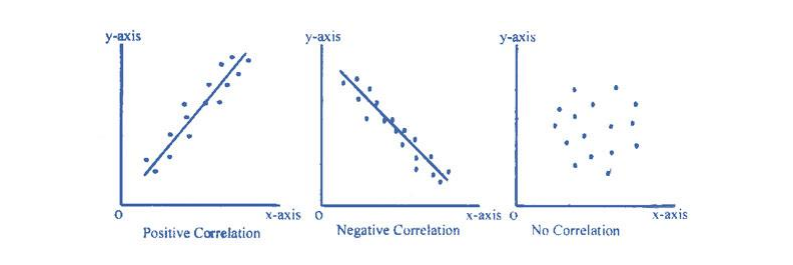Based on the change in proportion:

1. Linear: If the value of the amount of change in one variable tends to preserve a constant ratio to the amount of change in other variables, then the correlation is said to be linear. For Example, Whenever the price rises by 10%, then supply rises by 20%.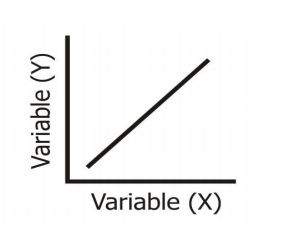2. Non-linear: If the value of the amount of change in one variable does not preserve a constant ratio to the amount of change in the other variables, then the it is said to be a Non-linear correlation. It is also known as the Curvilinear correlation. For Example, Whenever price rises by 10%, but supply rises sometimes by 20%, sometimes by 10%, and sometimes by 40%.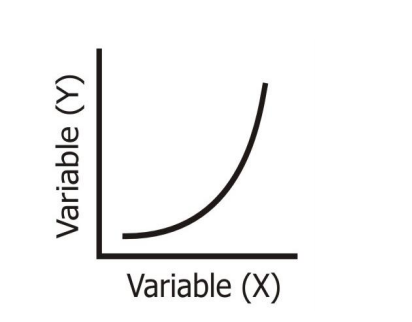Based on the number of variables studied:

1. Simple Correlation:  When we consider only two variables(Bivariate analysis) and check the correlation between only those variables, it is said to be a Simple Correlation. For example, Price and demand, Height and Weight, Income and consumption, etc.

2. Multiple Correlation:  When we consider more than three or three variables for correlation simultaneously, it is termed Multiple Correlation. For example, When we study the relationship between the yield of rice per hectare and both the amount of rainfall along with the number of fertilizers are used to find the relationship with rice production.

3. Partial Correlation:  When one or more variables are kept constant and the relationship is studied between the remaining variables, then it is termed Partial Corr. Study the relationship between 2 variables and assuming other variables are constant. For example, Relationship between rainfall and rice yields under constant temperature.

## How to Calculate Corr Coefficient Using Python?

Step-1: Import necessary Dependencies.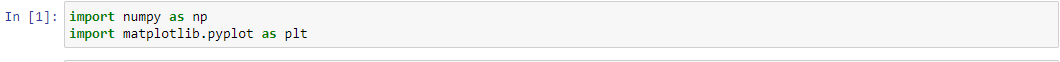Step-2: Calculate Pearson’s core coefficient using Numpy.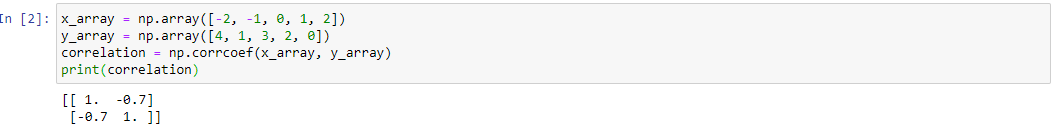## CONCLUSION

The output of the Numpy corrcoef() function is the correlation matrix, in which the diagonal entries give the correlation between a variable with itself and non-diagonal entries represent the corr between variables. This matrix is symmetric.

## End Notes

If you liked this and want to know more, go visit my other articles on Data Science and Machine Learning by clicking on the Link

Something not mentioned or want to share your thoughts? Feel free to comment below And I’ll get back to you.

Till then Stay Home, Stay Safe to prevent the spread of COVID-19, and Keep Learning!

## Chirag Goyal

Currently, I pursuing my Bachelor of Technology (B.Tech) in Computer Science and Engineering from the Indian Institute of Technology Jodhpur(IITJ). I am very enthusiastic about Machine learning, Deep Learning, and Artificial Intelligence.

The media shown in this article are not owned by Analytics Vidhya and is used at the Author’s discretion.